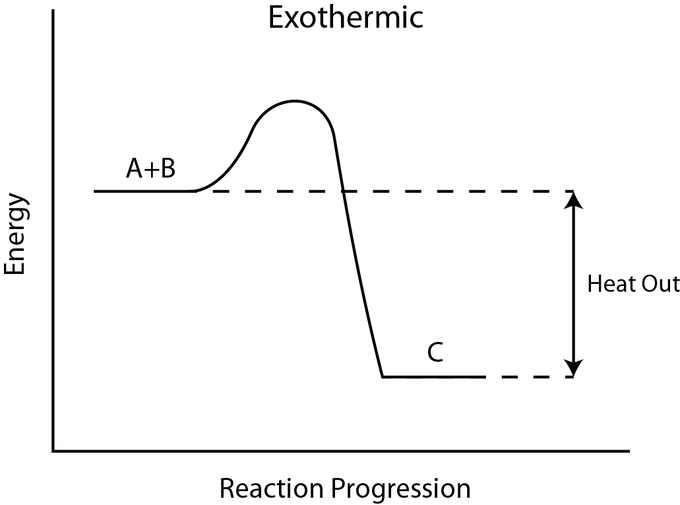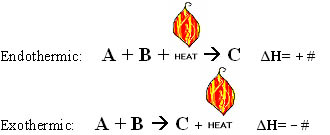Internal Energy and Enthalpy

The enthalpy of reaction measures the heat released/absorbed by a reaction that occurs at constant pressure.

Learning Objectives

Review enthalpy of reaction

Key Takeaways

Key Points

• At constant volume, the heat of reaction is equal to the change in the internal energy of the system.
• At constant pressure, the heat of reaction is equal to the enthalpy change of the system.
• Most chemical reactions occur at constant pressure, so enthalpy is more often used to measure heats of reaction than internal energy.

Key Terms

• enthalpy: In thermodynamics, a measure of the heat content of a chemical or physical system.
• internal energy: A property characteristic of the state of a thermodynamic system, the change in which is equal to the heat absorbed minus the work done by the system.
• first law of thermodynamics: Heat and work are forms of energy transfer; the internal energy of a closed system changes as heat and work are transferred into or out of it.

In thermodynamics, work (W) is defined as the process of an energy transfer from one system to another. The first law of thermodynamics states that the energy of a closed system is equal to the amount of heat supplied to the system minus the amount of work done by the system on its surroundings. The amount of energy for a closed system is written as follows:

$\Delta U = Q - W$

In this equation, U is the total energy of the system, Q is heat, and W is work. In chemical systems, the most common type of work is pressure-volume (PV) work, in which the volume of a gas changes. Substituting this in for work in the above equation, we can define the change in internal energy for a chemical system:

$\Delta U=Q-P\Delta V$

Internal Energy Change at Constant Volume

Let’s examine the internal energy change, $\Delta U$, at constant volume. At constant volume, $\Delta V=0$, the equation for the change in internal energy reduces to the following:

$\Delta U = Q_V$

The subscript V is added to Q to indicate that this is the heat transfer associated with a chemical process at constant volume. This internal energy is often very difficult to calculate in real life settings, though, because chemists tend to run their reactions in open flasks and beakers that allow gases to escape to the atmosphere. Therefore, volume is not held constant, and calculating $\Delta U$ becomes problematic. To correct for this, we introduce the concept of enthalpy, which is much more commonly used by chemists.

Standard Enthalpy of Reaction

The enthalpy of reaction is defined as the internal energy of the reaction system, plus the product of pressure and volume. It is given by:

$H=U+PV$

By adding the PV term, it becomes possible to measure a change in energy within a chemical system, even when that system does work on its surroundings. Most often, we are interested in the change in enthalpy of a given reaction, which can be expressed as follows:

$\Delta H = \Delta U +P\Delta V$

When you run a chemical reaction in a laboratory, the reaction occurs at constant pressure, because the atmospheric pressure around us is relatively constant. We will examine the change in enthalpy for a reaction at constant pressure, in order to see why enthalpy is such a useful concept for chemists.

Enthalpy of Reaction at Constant Pressure

Let’s look once again at the change in enthalpy for a given chemical process. It is given as follows:

$\Delta H=\Delta U + P\Delta V$

However, we also know that:

$\Delta U=Q-W=Q-P\Delta V$

Substituting to combine these two equations, we have:

$\Delta H=Q-P\Delta V+P \Delta V=Q_P$

Thus, at constant pressure, the change in enthalpy is simply equal to the heat released/absorbed by the reaction. Due to this relation, the change in enthalpy is often referred to simply as the “heat of reaction.”

Enthalpy: An explanation of why enthalpy can be viewed as “heat content” in a constant pressure system.

Exothermic and Endothermic Processes

Endothermic reactions absorb energy from the environment, while exothermic reactions release energy to the environment.

Learning Objectives

Distinguish between endothermic and exothermic reactions

Key Takeaways

Key Points

• All chemical reactions involve the transfer of energy.
• Endothermic processes require an input of energy to proceed and are signified by a positive change in enthalpy.
• Exothermic processes release energy upon completion, and are signified by a negative change in enthalpy.

Key Terms

• exothermic: Of a chemical reaction that releases energy in the form of heat.
• enthalpy: In thermodynamics, a measure of the heat content of a chemical or physical system.
• endothermic: Of a chemical reaction that absorbs heat energy from its surroundings.

All chemical processes are accompanied by energy changes. When a reaction proceeds, it either releases energy to, or absorbs energy from, its surroundings. In thermodynamics, these two types of reactions are classified as exothermic or endothermic, respectively. An easy way to remember the difference between these two reaction types is by their prefixes: endo- means to draw in, and exo- means to give off. We will explore these concepts in more detail after introducing the concept of enthalpy.

Enthalpy

Enthalpy (signified as H) is a measure of the total energy of a system and often expresses and simplifies energy transfer between systems. Since the total enthalpy of a system cannot be measured directly, we most often refer to the change in enthalpy for a particular chemical reaction. At constant pressure, the change in enthalpy is equal to the heat given off, or the heat absorbed, in a given chemical reaction:

$\Delta H=q_{rxn}$

Due to this relation, the change in enthalpy, $\Delta H$, is often referred to as the “heat of reaction.”

Exothermic Reactions

Exothermic reactions are reactions or processes that release energy, usually in the form of heat or light. In an exothermic reaction, energy is released because the total energy of the products is less than the total energy of the reactants. For this reason, the change in enthalpy, $\Delta H$, for an exothermic reaction will always be negative. In the presence of water, a strong acid will dissociate quickly and release heat, so it is an exothermic reaction.Exothermic reaction: In an exothermic reaction, the total energy of the products is less than the total energy of the reactants. Therefore, the change in enthalpy is negative, and heat is released to the surroundings.

Endothermic Reactions

Endothermic reactions are reactions that require external energy, usually in the form of heat, for the reaction to proceed. Since endothermic reactions draw in heat from their surroundings, they tend to cause their environments to cool down. They are also generally non-spontaneous, since endothermic reactions yield products that are higher in energy than the reactants. As such, the change in enthalpy for an endothermic reaction is always positive. In order to melt the ice cube, heat is required, so the process is endothermic.Endothermic reaction: In an endothermic reaction, the products are higher in energy than the reactants. Therefore, the change in enthalpy is positive, and heat is absorbed from the surroundings by the reaction.

Whether a reaction is endothermic or exothermic depends on the direction that it is going; some reactions are reversible, and when you revert the products back to reactants, the change in enthalpy is opposite.

Endothermic and exothermic reactions: Paul Andersen explains how heat can be absorbed in endothermic or released in exothermic reactions. An energy diagram can be used to show energy movements in these reactions and temperature can be used to measure them macroscopically.

Thermochemical Equations

Thermochemical equations are chemical equations which include the enthalpy change of the reaction, $\Delta H_{rxn}$.

Learning Objectives

Give examples of thermochemical equations

Key Takeaways

Key Points

• If $\Delta H$ is negative, the reaction is exothermic; if $\Delta H$ is positive, the reaction is endothermic.
• Thermochemical equations have the general form: $A+B\rightarrow C, \Delta H=(\pm n)$.
• The value of enthalpy is dependent on the reaction conditions, as well as the concentration of reactants and products.

Key Terms

• thermochemical equation: A special equation type, denoting the overall change in energy.
• enthalpy: In thermodynamics, a measure of the heat content of a chemical or physical system.
• Hess’s law: States that the enthalpy change for a reaction is the same whether it occurs in one step or in a series of steps.

Enthalpy (H) is a measure of the energy in a system, and the change in enthalpy is denoted by $\Delta H$. Since enthalpy is a state function, the value of $\Delta H$ is independent of the path taken by the reactions to reach the products. Values of $\Delta H$ can be determined experimentally under standard conditions.

A thermochemical equation is a balanced stoichiometric chemical equation which includes the enthalpy change. The equations take the form: $A+B\rightarrow C,\: \Delta H =(\pm n)$

Thermochemical Equations for Endothermic Reactions

The sign of the $\Delta H$ value indicates whether or not the system is endothermic or exothermic. In an endothermic system, the $\Delta H$ value is positive, so the reaction absorbs heat into the system. The equation takes the form:

$\text{heat}+A+B\rightarrow C,\;\Delta H=+$

Notice that in an endothermic reaction like the one depicted above, we can think of heat as being a reactant, just like A and B.

Thermochemical Equations for Exothermic Reactions

In an exothermic system, the $\Delta H$ value is negative, so heat is given off by the reaction. The equation takes the form:

$A + B \rightarrow C + \text{heat},\: \Delta H = -$

Notice that here, we can think of heat as being a product in the reaction.

$\Delta H$ is dependent on both the phase (solid, liquid, or gas) as well as the molar ratios of the reactants and products. Therefore, all thermochemical equations must be stoichiometrically balanced. This becomes important once we begin working with Hess’s law.Thermochemical equations: Thermochemical equations can describe endothermic or exothermic reactions.